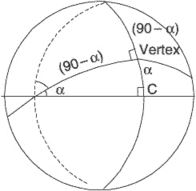# vertex

(redirected from vertex cordis)
Also found in: Dictionary, Thesaurus, Medical.

## vertex

1. Maths
a. the point opposite the base of a figure
b. the point of intersection of two sides of a plane figure or angle
c. the point of intersection of a pencil of lines or three or more planes of a solid figure
2. Astronomy a point in the sky towards which a star stream appears to move
3. Anatomy the crown of the head
Collins Discovery Encyclopedia, 1st edition © HarperCollins Publishers 2005

## Vertex

The highest point of a structure; the apex or summit.

## Vertex

(religion, spiritualism, and occult)

In geometry, a vertex is the pivot point of an angle. In astrology, the vertex is the point in a horoscope where the prime vertical intersects the ecliptic in the west. The antivertex is the corresponding point in the east. The vertex was discovered/invented by L. Edward Johndro and elaborated upon by Charles Jayne. The point where the vertex falls in a chart is said to be the most fated (i.e., least amenable to conscious choices) part of the horoscope. All major astrological chart-casting software programs allow one to calculate the vertex.

The following article is from The Great Soviet Encyclopedia (1979). It might be outdated or ideologically biased.

## Vertex

the point on the celestial sphere toward which the peculiar motions of stars are predominantly directed. There are two such points, diametrically opposed on the celestial sphere, with the galactic coordinates l = 344°, b -1° and l = 164°, b = 1°.

## vertex

[′vər‚teks]
(astronomy)
The highest point that a celestial body attains.
On a great circle, that point that is closest to a pole.
(mathematics)
For a polygon or polyhedron, any of those finitely many points which together with line segments or plane pieces determine the figure or solid.
The common point at which the two sides of an angle intersect.
The fixed point through which pass all the elements of a cone or conical surface.
An intersection of a conic with one of its axes of symmetry.
A member of the set of points that are connected by the edges. Also known as node.
For a simplex, one of the finite set of points on which a simplex is based.
(optics)
One of the points where the surface of a lens intersects the optical axis.
McGraw-Hill Dictionary of Scientific & Technical Terms, 6E, Copyright © 2003 by The McGraw-Hill Companies, Inc.

## vertexEvery great circle intersects two points, 180° apart. These points are 90° away from the longitude of the vertices. The angle at which the great circle intersects the equator is equal to the co-latitude of the vertices. The purpose of determining position of the vertex is to facilitate calculation of intermediate position along the great circle track between which short rhumb-line courses can be steered. Based on the fact that the great circle track intersects the meridian of the vertex at 90°, Napier's rule can be used to solve the successive right angles to obtain intermediate positions.
i. The last fix adapted on the arrival speed segment. Normally, it will be the outer marker of the runway in use. However, it may be the actual threshold or another suitable common point on the approach path for the particular runway configuration.
ii. In astronavigation, the vertex of a great circle is the point at which the great circle is nearest the geographic pole—that is, the point at which it reaches the maximum latitude. Every great circle has two vertices (one in each hemisphere). In the solution of great-circle sailing problems, only the vertex nearest the arc forming the great-circle track is considered.